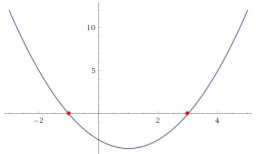# Intersections 25141

The quadratic function has the formula y = x²-2x-3. Sketch a graph of this function. Find the intersections with the axes. Find the vertex coordinates.

y0 =  -3
x1 =  3
x2 =  -1
x3 =  1
y3 =  -4

### Step-by-step explanation:Did you find an error or inaccuracy? Feel free to write us. Thank you!

Tips for related online calculators
Are you looking for help with calculating roots of a quadratic equation?